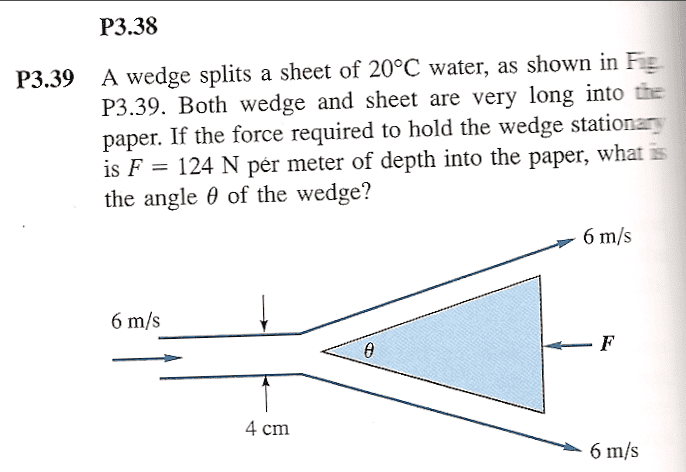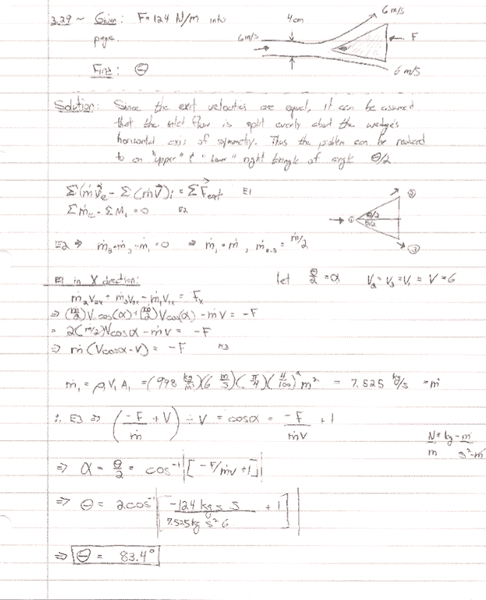# Fluids Cons of Momentum

## Homework Statement## Homework Equations

$$\sum(\dot{m}\vec{v})_{exit}-\sum(\dot{m}\vec{v})_{inlet}=\sum F_{ext}$$

I only know that this is cons of momentum because my prof told us. I am having a hard time visualizing how to incorporate THETA into the above equation since it is not a nice right triangle.

I am thinking that since the exit velocities are equal, than the inlet flow must be being split about the wedges horizontal axis of symmetry.

Thus, I think I can divide the wedge into an "upper" and "lower" right triangle whose angle wrt to the horizontal is $\frac{\theta}{2}$.

Then I can resolve the momentum eq into Cartesian coordinates.

Sound good?

Mentor
Sounds lovely!

Nice! I guess that what is confusing me now, is why they gave me
force/unit length into page

But I am just going to start plugging in and see what happens.

Mentor
I guess that what is confusing me now, is why they gave me
force/unit length into page
Consider it to be an arbitrarily wide sheet of water. Use momentum per unit length as well.

Consider it to be an arbitrarily wide sheet of water. Use momentum per unit length as well.

Okay, so the m-1 just drops out anyway, and this problem reduces to regular cons of mom

Mentor
Yep.

Thanks! 83.4 degrees sounds reasonable I thinkMentor
83.4 degrees sounds reasonable I think
Show how you got that answer.Mentor
Rethink your result for m, the mass flow rate. You want it to be mass flow per unit width (or depth).

I am not sure that I follow. Errr.... Okay. So that inlet with the 4 cm dimension is NOT a PIPE....right?

It is a "sheet" with area 4cm*WIDTH. Thus my, mass flow rate should be, with h=4cm:

$$\dot{m}=\rho V (h*W)\Rightarrow \frac{\dot{m}}{W}=\rho Vh$$

Am I with you now?

Mentor
Now you're cooking.

Typical 'not-paying-attention' mistake. Perhaps I should turn off Band of Brothers while I do my studies?